Diffusion models are a new type of generative models that are flexible enough to learn any arbitrarily complex data distribution while tractable to analytically evaluate the distribution. It has been shown recently that diffusion models can generate high-quality images and the performance is competitive to SOTA GAN.

[Updated on 2021-09-19: Highly recommend this blog post on score-based generative modeling by Yang Song (author of several key papers in the references)].

So far, I’ve written about three types of generative models, GAN, VAE, and Flow-based models. They have shown great success in generating high-quality samples, but each has some limitations of its own. GAN models are known for potentially unstable training and less diversity in generation due to their adversarial training nature. VAE relies on a surrogate loss. Flow models have to use specialized architectures to construct reversible transform.

Diffusion models are inspired by non-equilibrium thermodynamics. They define a Markov chain of diffusion steps to slowly add random noise to data and then learn to reverse the diffusion process to construct desired data samples from the noise. Unlike VAE or flow models, diffusion models are learned with a fixed procedure and the latent variable has high dimensionality (same as the original data).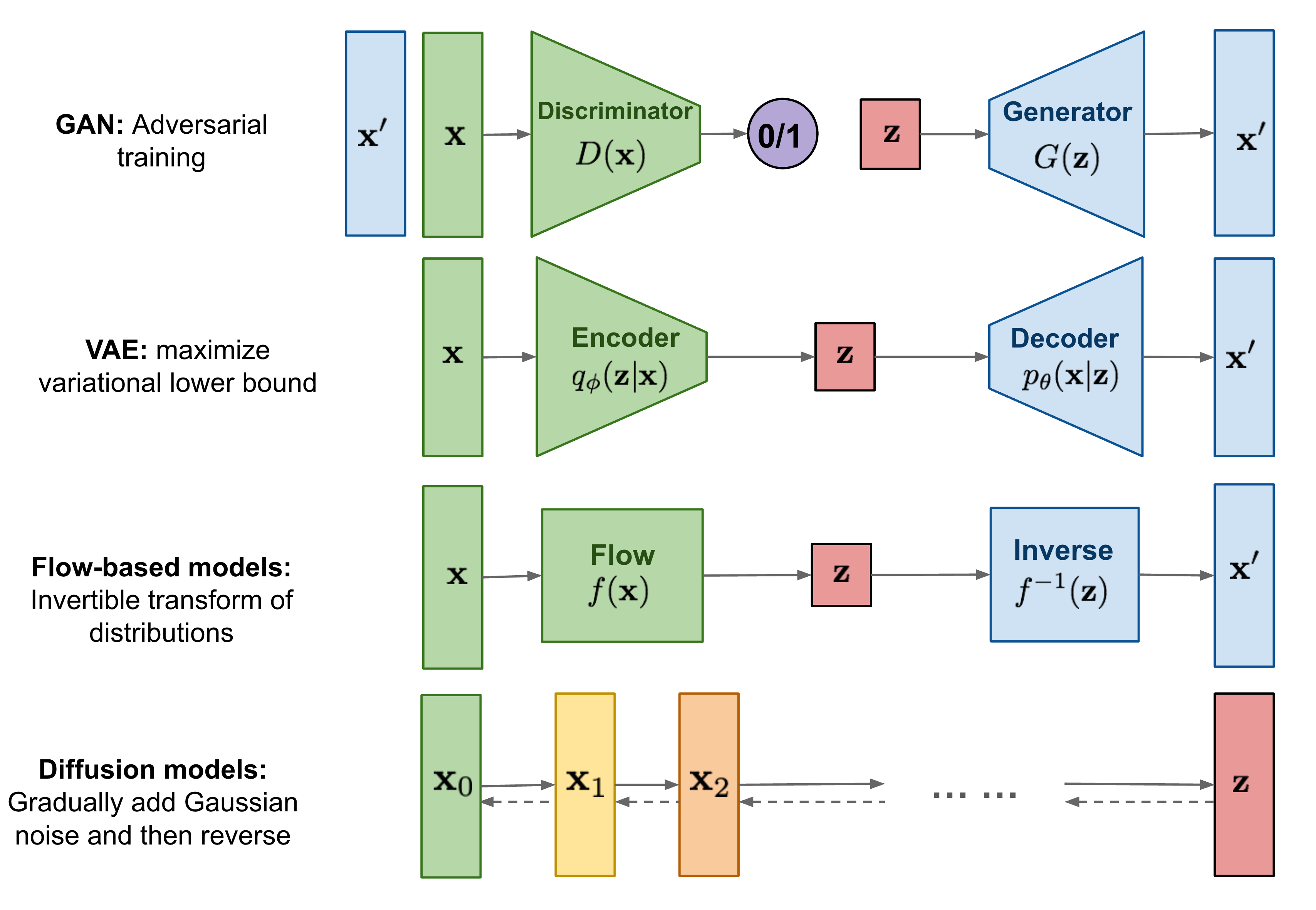Fig. 1. Overview of different types of generative models.

What are Diffusion Models?

Several diffusion-based generative models have been proposed with similar ideas underneath, including diffusion probabilistic models (Sohl-Dickstein et al., 2015), noise-conditioned score network (NCSN; Yang & Ermon, 2019), and denoising diffusion probabilistic models (DDPM; Ho et al. 2020).

Forward diffusion process

Given a data point sampled from a real data distribution $$\mathbf{x}_0 \sim q(\mathbf{x})$$, let us define a forward diffusion process in which we add small amount of Gaussian noise to the sample in $$T$$ steps, producing a sequence of noisy samples $$\mathbf{x}_1, \dots, \mathbf{x}_T$$. The step sizes are controlled by a variance schedule $$\{\beta_t \in (0, 1)\}_{t=1}^t$$.

$q(\mathbf{x}_t \vert \mathbf{x}_{t-1}) = \mathcal{N}(\mathbf{x}_t; \sqrt{1 - \beta_t} \mathbf{x}_{t-1}, \beta_t\mathbf{I}) \quad q(\mathbf{x}_{1:T} \vert \mathbf{x}_0) = \prod^T_{t=1} q(\mathbf{x}_t \vert \mathbf{x}_{t-1})$

The data sample $$\mathbf{x}_0$$ gradually loses its distinguishable features as the step $$t$$ becomes larger. Eventually when $$T \to \infty$$, $$\mathbf{x}_T$$ is equivalent to an isotropic Gaussian distribution.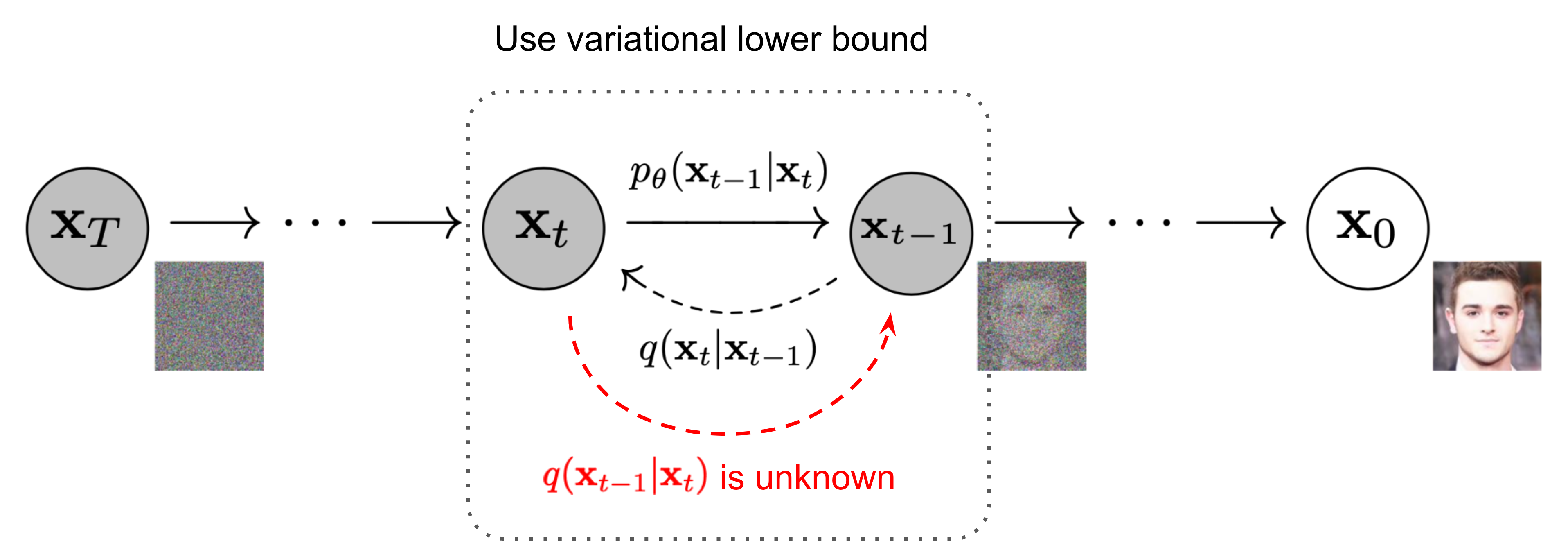Fig. 2. The Markov chain of forward (reverse) diffusion process of generating a sample by slowly adding (removing) noise. (Image source: Ho et al. 2020 with a few additional annotations)

A nice property of the above process is that we can sample $$\mathbf{x}_t$$ at any arbitrary time step $$t$$ in a closed form using reparameterization trick. Let $$\alpha_t = 1 - \beta_t$$ and $$\bar{\alpha}_t = \prod_{i=1}^T \alpha_i$$:

\begin{aligned} \mathbf{x}_t &= \sqrt{\alpha_t}\mathbf{x}_{t-1} + \sqrt{1 - \alpha_t}\mathbf{z}_{t-1} & \text{ ;where } \mathbf{z}_{t-1}, \mathbf{z}_{t-2}, \dots \sim \mathcal{N}(\mathbf{0}, \mathbf{I}) \\ &= \sqrt{\alpha_t \alpha_{t-1}} \mathbf{x}_{t-2} + \sqrt{1 - \alpha_t \alpha_{t-1}} \bar{\mathbf{z}}_{t-2} & \text{ ;where } \bar{\mathbf{z}}_{t-2} \text{ merges two Gaussians (*).} \\ &= \dots \\ &= \sqrt{\bar{\alpha}_t}\mathbf{x}_0 + \sqrt{1 - \bar{\alpha}_t}\mathbf{z} \\ q(\mathbf{x}_t \vert \mathbf{x}_0) &= \mathcal{N}(\mathbf{x}_t; \sqrt{\bar{\alpha}_t} \mathbf{x}_0, (1 - \bar{\alpha}_t)\mathbf{I}) \end{aligned}

(*) Recall that when we merge two Gaussians with different variance, $$\mathcal{N}(\mathbf{0}, \sigma_1^2\mathbf{I})$$ and $$\mathcal{N}(\mathbf{0}, \sigma_2^2\mathbf{I})$$, the new distribution is $$\mathcal{N}(\mathbf{0}, (\sigma_1^2 + \sigma_2^2)\mathbf{I})$$. Here the merged standard deviation is $$\sqrt{(1 - \alpha_t) + \alpha_t (1-\alpha_{t-1})} = \sqrt{1 - \alpha_t\alpha_{t-1}}$$.

Usually, we can afford a larger update step when the sample gets noisier, so $$\beta_1 < \beta_2 < \dots < \beta_T$$ and therefore $$\bar{\alpha}_1 > \dots > \bar{\alpha}_T$$.

Connection with stochastic gradient Langevin dynamics

Langevin dynamics is a concept from physics, developed for statistically modeling molecular systems. Combined with stochastic gradient descent, stochastic gradient Langevin dynamics (Welling & Teh 2011) can produce samples from a probability density $$p(\mathbf{x})$$ using only the gradients $$\nabla_\mathbf{x} \log p(\mathbf{x})$$ in a Markov chain of updates:

$\mathbf{x}_t = \mathbf{x}_{t-1} + \frac{\epsilon}{2} \nabla_\mathbf{x} p(\mathbf{x}_{t-1}) + \sqrt{\epsilon} \mathbf{z}_t ,\quad\text{where } \mathbf{z}_t \sim \mathcal{N}(\mathbf{0}, \mathbf{I})$

where $$\epsilon$$ is the step size. When $$T \to \infty, \epsilon \to 0$$, $$\mathbf{x}_T$$ equals to the true probability density $$p(\mathbf{x})$$.

Compared to standard SGD, stochastic gradient Langevin dynamics injects Gaussian noise into the parameter updates to avoid collapses into local minima.

Reverse diffusion process

If we can reverse the above process and sample from $$q(\mathbf{x}_{t-1} \vert \mathbf{x}_t)$$, we will be able to recreate the true sample from a Gaussian noise input, $$\mathbf{x}_T \sim \mathcal{N}(\mathbf{0}, \mathbf{I})$$. Note that if $$\beta_t$$ is small enough, $$q(\mathbf{x}_{t-1} \vert \mathbf{x}_t)$$ will also be Gaussian. Unfortunately, we cannot easily estimate $$q(\mathbf{x}_{t-1} \vert \mathbf{x}_t)$$ because it needs to use the entire dataset and therefore we need to learn a model $$p_\theta$$ to approximate these conditional probabilities in order to run the reverse diffusion process.

$p_\theta(\mathbf{x}_{0:T}) = p(\mathbf{x}_T) \prod^T_{t=1} p_\theta(\mathbf{x}_{t-1} \vert \mathbf{x}_t) \quad p_\theta(\mathbf{x}_{t-1} \vert \mathbf{x}_t) = \mathcal{N}(\mathbf{x}_{t-1}; \boldsymbol{\mu}_\theta(\mathbf{x}_t, t), \boldsymbol{\Sigma}_\theta(\mathbf{x}_t, t))$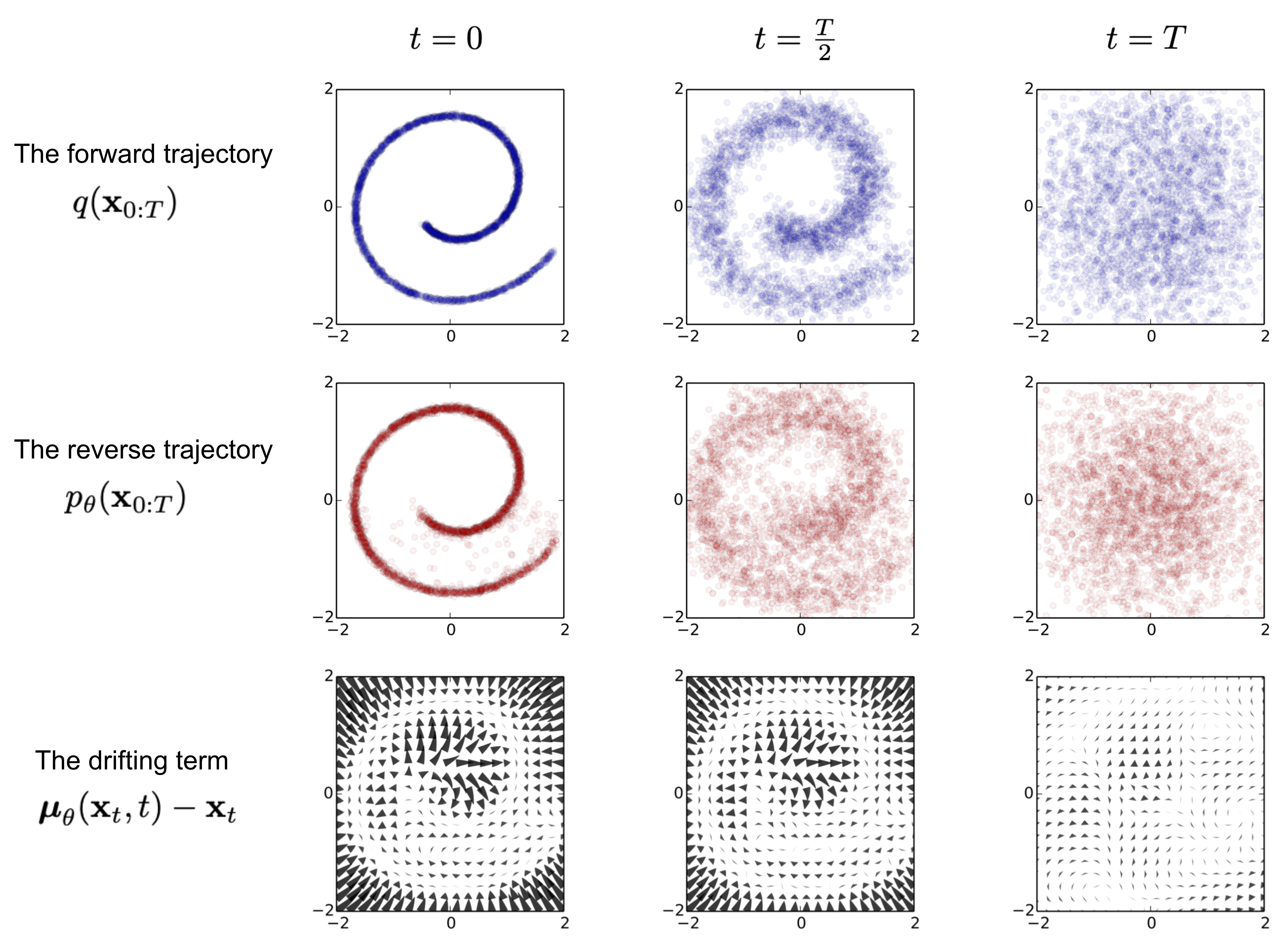Fig. 3. An example of training a diffusion model for modeling a 2D swiss roll data. (Image source: Sohl-Dickstein et al., 2015)

It is noteworthy that the reverse conditional probability is tractable when conditioned on $$\mathbf{x}_0$$:

$q(\mathbf{x}_{t-1} \vert \mathbf{x}_t, \mathbf{x}_0) = \mathcal{N}(\mathbf{x}_{t-1}; \color{blue}{\tilde{\boldsymbol{\mu}}}(\mathbf{x}_t, \mathbf{x}_0), \color{red}{\tilde{\beta}_t} \mathbf{I})$

Using Bayes’ rule, we have:

\begin{aligned} q(\mathbf{x}_{t-1} \vert \mathbf{x}_t, \mathbf{x}_0) &= q(\mathbf{x}_t \vert \mathbf{x}_{t-1}, \mathbf{x}_0) \frac{ q(\mathbf{x}_{t-1} \vert \mathbf{x}_0) }{ q(\mathbf{x}_t \vert \mathbf{x}_0) } \\ &\propto \exp \Big(-\frac{1}{2} \big(\frac{(\mathbf{x}_t - \sqrt{\alpha_t} \mathbf{x}_{t-1})^2}{\beta_t} + \frac{(\mathbf{x}_{t-1} - \sqrt{\bar{\alpha}_{t-1}} \mathbf{x}_0)^2}{1-\bar{\alpha}_{t-1}} - \frac{(\mathbf{x}_t - \sqrt{\bar{\alpha}_t} \mathbf{x}_0)^2}{1-\bar{\alpha}_t} \big) \Big) \\ &= \exp\Big( -\frac{1}{2} \big( \color{red}{(\frac{\alpha_t}{\beta_t} + \frac{1}{1 - \bar{\alpha}_{t-1}})} \mathbf{x}_{t-1}^2 - \color{blue}{(\frac{2\sqrt{\alpha_t}}{\beta_t} \mathbf{x}_t + \frac{2\sqrt{\bar{\alpha}_t}}{1 - \bar{\alpha}_t} \mathbf{x}_0)} \mathbf{x}_{t-1} + C(\mathbf{x}_t, \mathbf{x}_0) \big) \Big) \end{aligned}

where $$C(\mathbf{x}_t, \mathbf{x}_0)$$ is some function not involving $$\mathbf{x}_{t-1}$$ and details are omitted. Following the standard Gaussian density function, the mean and variance can be parameterized as follows:

\begin{aligned} \tilde{\beta}_t &= 1/(\frac{\alpha_t}{\beta_t} + \frac{1}{1 - \bar{\alpha}_{t-1}}) = \frac{1 - \bar{\alpha}_{t-1}}{1 - \bar{\alpha}_t} \cdot \beta_t \\ \tilde{\boldsymbol{\mu}}_t (\mathbf{x}_t, \mathbf{x}_0) &= (\frac{\sqrt{\alpha_t}}{\beta_t} \mathbf{x}_t + \frac{\sqrt{\bar{\alpha}_t}}{1 - \bar{\alpha}_t} \mathbf{x}_0)/(\frac{\alpha_t}{\beta_t} + \frac{1}{1 - \bar{\alpha}_{t-1}}) = \frac{\sqrt{\alpha_t}(1 - \bar{\alpha}_{t-1})}{1 - \bar{\alpha}_t} \mathbf{x}_t + \frac{\sqrt{\bar{\alpha}_{t-1}}\beta_t}{1 - \bar{\alpha}_t} \mathbf{x}_0\\ \end{aligned}

Thanks to the nice property, we can represent $$\mathbf{x}_0 = \frac{1}{\sqrt{\bar{\alpha}_t}}(\mathbf{x}_t - \sqrt{1 - \bar{\alpha}_t}\mathbf{z}_t)$$ and plug it into the above equation and obtain:

\begin{aligned} \tilde{\boldsymbol{\mu}}_t &= \frac{\sqrt{\alpha_t}(1 - \bar{\alpha}_{t-1})}{1 - \bar{\alpha}_t} \mathbf{x}_t + \frac{\sqrt{\bar{\alpha}_{t-1}}\beta_t}{1 - \bar{\alpha}_t} \frac{1}{\sqrt{\bar{\alpha}_t}}(\mathbf{x}_t - \sqrt{1 - \bar{\alpha}_t}\mathbf{z}_t) \\ &= \color{cyan}{\frac{1}{\sqrt{\alpha_t}} \Big( \mathbf{x}_t - \frac{\beta_t}{\sqrt{1 - \bar{\alpha}_t}} \mathbf{z}_t \Big)} \end{aligned}

As demonstrated in Fig. 2., such a setup is very similar to VAE and thus we can use the variational lower bound to optimize the negative log-likelihood.

\begin{aligned} - \log p_\theta(\mathbf{x}_0) &\leq - \log p_\theta(\mathbf{x}_0) + D_\text{KL}(q(\mathbf{x}_{1:T}\vert\mathbf{x}_0) \| p_\theta(\mathbf{x}_{1:T}\vert\mathbf{x}_0) ) \\ &= -\log p_\theta(\mathbf{x}_0) + \mathbb{E}_{\mathbf{x}_{1:T}\sim q(\mathbf{x}_{1:T} \vert \mathbf{x}_0)} \Big[ \log\frac{q(\mathbf{x}_{1:T}\vert\mathbf{x}_0)}{p_\theta(\mathbf{x}_{0:T}) / p_\theta(\mathbf{x}_0)} \Big] \\ &= -\log p_\theta(\mathbf{x}_0) + \mathbb{E}_q \Big[ \log\frac{q(\mathbf{x}_{1:T}\vert\mathbf{x}_0)}{p_\theta(\mathbf{x}_{0:T})} + \log p_\theta(\mathbf{x}_0) \Big] \\ &= \mathbb{E}_q \Big[ \log \frac{q(\mathbf{x}_{1:T}\vert\mathbf{x}_0)}{p_\theta(\mathbf{x}_{0:T})} \Big] \\ \text{Let }L_\text{VLB} &= \mathbb{E}_{q(\mathbf{x}_{0:T})} \Big[ \log \frac{q(\mathbf{x}_{1:T}\vert\mathbf{x}_0)}{p_\theta(\mathbf{x}_{0:T})} \Big] \geq - \mathbb{E}_{q(\mathbf{x}_0)} \log p_\theta(\mathbf{x}_0) \end{aligned}

It is also straightforward to get the same result using Jensen’s inequality. Say we want to minimize the cross entropy as the learning objective,

\begin{aligned} L_\text{CE} &= - \mathbb{E}_{q(\mathbf{x}_0)} \log p_\theta(\mathbf{x}_0) \\ &= - \mathbb{E}_{q(\mathbf{x}_0)} \log \Big( \int p_\theta(\mathbf{x}_{0:T}) d\mathbf{x}_{1:T} \Big) \\ &= - \mathbb{E}_{q(\mathbf{x}_0)} \log \Big( \int q(\mathbf{x}_{1:T} \vert \mathbf{x}_0) \frac{p_\theta(\mathbf{x}_{0:T})}{q(\mathbf{x}_{1:T} \vert \mathbf{x}_{0})} d\mathbf{x}_{1:T} \Big) \\ &= - \mathbb{E}_{q(\mathbf{x}_0)} \log \Big( \mathbb{E}_{q(\mathbf{x}_{1:T} \vert \mathbf{x}_0)} \frac{p_\theta(\mathbf{x}_{0:T})}{q(\mathbf{x}_{1:T} \vert \mathbf{x}_{0})} \Big) \\ &\leq - \mathbb{E}_{q(\mathbf{x}_{0:T})} \log \frac{p_\theta(\mathbf{x}_{0:T})}{q(\mathbf{x}_{1:T} \vert \mathbf{x}_{0})} \\ &= \mathbb{E}_{q(\mathbf{x}_{0:T})}\Big[\log \frac{q(\mathbf{x}_{1:T} \vert \mathbf{x}_{0})}{p_\theta(\mathbf{x}_{0:T})} \Big] = L_\text{VLB} \end{aligned}

To convert each term in the equation to be analytically computable, the objective can be further rewritten to be a combination of several KL-divergence and entropy terms (See the detailed step-by-step process in Appendix B in Sohl-Dickstein et al., 2015):

\begin{aligned} L_\text{VLB} &= \mathbb{E}_{q(\mathbf{x}_{0:T})} \Big[ \log\frac{q(\mathbf{x}_{1:T}\vert\mathbf{x}_0)}{p_\theta(\mathbf{x}_{0:T})} \Big] \\ &= \mathbb{E}_q \Big[ \log\frac{\prod_{t=1}^T q(\mathbf{x}_t\vert\mathbf{x}_{t-1})}{ p_\theta(\mathbf{x}_T) \prod_{t=1}^T p_\theta(\mathbf{x}_{t-1} \vert\mathbf{x}_t) } \Big] \\ &= \mathbb{E}_q \Big[ -\log p_\theta(\mathbf{x}_T) + \sum_{t=1}^T \log \frac{q(\mathbf{x}_t\vert\mathbf{x}_{t-1})}{p_\theta(\mathbf{x}_{t-1} \vert\mathbf{x}_t)} \Big] \\ &= \mathbb{E}_q \Big[ -\log p_\theta(\mathbf{x}_T) + \sum_{t=2}^T \log \frac{q(\mathbf{x}_t\vert\mathbf{x}_{t-1})}{p_\theta(\mathbf{x}_{t-1} \vert\mathbf{x}_t)} + \log\frac{q(\mathbf{x}_1 \vert \mathbf{x}_0)}{p_\theta(\mathbf{x}_0 \vert \mathbf{x}_1)} \Big] \\ &= \mathbb{E}_q \Big[ -\log p_\theta(\mathbf{x}_T) + \sum_{t=2}^T \log \Big( \frac{q(\mathbf{x}_{t-1} \vert \mathbf{x}_t, \mathbf{x}_0)}{p_\theta(\mathbf{x}_{t-1} \vert\mathbf{x}_t)}\cdot \frac{q(\mathbf{x}_t \vert \mathbf{x}_0)}{q(\mathbf{x}_{t-1}\vert\mathbf{x}_0)} \Big) + \log \frac{q(\mathbf{x}_1 \vert \mathbf{x}_0)}{p_\theta(\mathbf{x}_0 \vert \mathbf{x}_1)} \Big] \\ &= \mathbb{E}_q \Big[ -\log p_\theta(\mathbf{x}_T) + \sum_{t=2}^T \log \frac{q(\mathbf{x}_{t-1} \vert \mathbf{x}_t, \mathbf{x}_0)}{p_\theta(\mathbf{x}_{t-1} \vert\mathbf{x}_t)} + \sum_{t=2}^T \log \frac{q(\mathbf{x}_t \vert \mathbf{x}_0)}{q(\mathbf{x}_{t-1} \vert \mathbf{x}_0)} + \log\frac{q(\mathbf{x}_1 \vert \mathbf{x}_0)}{p_\theta(\mathbf{x}_0 \vert \mathbf{x}_1)} \Big] \\ &= \mathbb{E}_q \Big[ -\log p_\theta(\mathbf{x}_T) + \sum_{t=2}^T \log \frac{q(\mathbf{x}_{t-1} \vert \mathbf{x}_t, \mathbf{x}_0)}{p_\theta(\mathbf{x}_{t-1} \vert\mathbf{x}_t)} + \log\frac{q(\mathbf{x}_T \vert \mathbf{x}_0)}{q(\mathbf{x}_1 \vert \mathbf{x}_0)} + \log \frac{q(\mathbf{x}_1 \vert \mathbf{x}_0)}{p_\theta(\mathbf{x}_0 \vert \mathbf{x}_1)} \Big]\\ &= \mathbb{E}_q \Big[ \log\frac{q(\mathbf{x}_T \vert \mathbf{x}_0)}{p_\theta(\mathbf{x}_T)} + \sum_{t=2}^T \log \frac{q(\mathbf{x}_{t-1} \vert \mathbf{x}_t, \mathbf{x}_0)}{p_\theta(\mathbf{x}_{t-1} \vert\mathbf{x}_t)} - \log p_\theta(\mathbf{x}_0 \vert \mathbf{x}_1) \Big] \\ &= \mathbb{E}_q [\underbrace{D_\text{KL}(q(\mathbf{x}_T \vert \mathbf{x}_0) \parallel p_\theta(\mathbf{x}_T))}_{L_T} + \sum_{t=2}^T \underbrace{D_\text{KL}(q(\mathbf{x}_{t-1} \vert \mathbf{x}_t, \mathbf{x}_0) \parallel p_\theta(\mathbf{x}_{t-1} \vert\mathbf{x}_t))}_{L_{t-1}} \underbrace{- \log p_\theta(\mathbf{x}_0 \vert \mathbf{x}_1)}_{L_0} ] \end{aligned}

Let’s label each component in the variational lower bound loss separately:

\begin{aligned} L_\text{VLB} &= L_T + L_{T-1} + \dots + L_0 \\ \text{where } L_T &= D_\text{KL}(q(\mathbf{x}_T \vert \mathbf{x}_0) \parallel p_\theta(\mathbf{x}_T)) \\ L_t &= D_\text{KL}(q(\mathbf{x}_t \vert \mathbf{x}_{t+1}, \mathbf{x}_0) \parallel p_\theta(\mathbf{x}_t \vert\mathbf{x}_{t+1})) \text{ for }1 \leq t \leq T-1 \\ L_0 &= - \log p_\theta(\mathbf{x}_0 \vert \mathbf{x}_1) \end{aligned}

Every KL term in $$L_\text{VLB}$$ (except for $$L_0$$) compares two Gaussian distributions and therefore they can be computed in closed form. $$L_T$$ is constant and can be ignored during training because $$q$$ has no learnable parameters and $$\mathbf{x}_T$$ is a Gaussian noise. Ho et al. 2020 models $$L_0$$ using a separate discrete decoder derived from $$\mathcal{N}(\mathbf{x}_0; \boldsymbol{\mu}_\theta(\mathbf{x}_1, 1), \boldsymbol{\Sigma}_\theta(\mathbf{x}_1, 1))$$.

Parameterization of $$L_t$$ for Training Loss

Recall that we need to learn a neural network to approximate the conditioned probability distributions in the reverse diffusion process, $$p_\theta(\mathbf{x}_{t-1} \vert \mathbf{x}_t) = \mathcal{N}(\mathbf{x}_{t-1}; \boldsymbol{\mu}_\theta(\mathbf{x}_t, t), \boldsymbol{\Sigma}_\theta(\mathbf{x}_t, t))$$. We would like to train $$\boldsymbol{\mu}_\theta$$ to predict $$\tilde{\boldsymbol{\mu}}_t = \frac{1}{\sqrt{\alpha_t}} \Big( \mathbf{x}_t - \frac{\beta_t}{\sqrt{1 - \bar{\alpha}_t}} \mathbf{z}_t \Big)$$. Because $$\mathbf{x}_t$$ is available as input at training time, we can reparameterize the Gaussian noise term instead to make it predict $$\mathbf{z}_t$$ from the input $$\mathbf{x}_t$$ at time step $$t$$:

\begin{aligned} \boldsymbol{\mu}_\theta(\mathbf{x}_t, t) &= \color{cyan}{\frac{1}{\sqrt{\alpha_t}} \Big( \mathbf{x}_t - \frac{\beta_t}{\sqrt{1 - \bar{\alpha}_t}} \mathbf{z}_\theta(\mathbf{x}_t, t) \Big)} \\ \text{Thus }\mathbf{x}_{t-1} &= \mathcal{N}(\mathbf{x}_{t-1}; \frac{1}{\sqrt{\alpha_t}} \Big( \mathbf{x}_t - \frac{\beta_t}{\sqrt{1 - \bar{\alpha}_t}} \mathbf{z}_\theta(\mathbf{x}_t, t) \Big), \boldsymbol{\Sigma}_\theta(\mathbf{x}_t, t)) \end{aligned}

The loss term $$L_t$$ is parameterized to minimize the difference from $$\tilde{\boldsymbol{\mu}}$$ :

\begin{aligned} L_t &= \mathbb{E}_{\mathbf{x}_0, \mathbf{z}} \Big[\frac{1}{2 \| \boldsymbol{\Sigma}_\theta(\mathbf{x}_t, t) \|^2_2} \| \color{blue}{\tilde{\boldsymbol{\mu}}_t(\mathbf{x}_t, \mathbf{x}_0)} - \color{green}{\boldsymbol{\mu}_\theta(\mathbf{x}_t, t)} \|^2 \Big] \\ &= \mathbb{E}_{\mathbf{x}_0, \mathbf{z}} \Big[\frac{1}{2 \|\boldsymbol{\Sigma}_\theta \|^2_2} \| \color{blue}{\frac{1}{\sqrt{\alpha_t}} \Big( \mathbf{x}_t - \frac{\beta_t}{\sqrt{1 - \bar{\alpha}_t}} \mathbf{z}_t \Big)} - \color{green}{\frac{1}{\sqrt{\alpha_t}} \Big( \mathbf{x}_t - \frac{\beta_t}{\sqrt{1 - \bar{\alpha}_t}} \boldsymbol{\mathbf{z}}_\theta(\mathbf{x}_t, t) \Big)} \|^2 \Big] \\ &= \mathbb{E}_{\mathbf{x}_0, \mathbf{z}} \Big[\frac{ \beta_t^2 }{2 \alpha_t (1 - \bar{\alpha}_t) \| \boldsymbol{\Sigma}_\theta \|^2_2} \|\mathbf{z}_t - \mathbf{z}_\theta(\mathbf{x}_t, t)\|^2 \Big] \\ &= \mathbb{E}_{\mathbf{x}_0, \mathbf{z}} \Big[\frac{ \beta_t^2 }{2 \alpha_t (1 - \bar{\alpha}_t) \| \boldsymbol{\Sigma}_\theta \|^2_2} \|\mathbf{z}_t - \mathbf{z}_\theta(\sqrt{\bar{\alpha}_t}\mathbf{x}_0 + \sqrt{1 - \bar{\alpha}_t}\mathbf{z}_t, t)\|^2 \Big] \end{aligned}

Simplification

Empirically, Ho et al. (2020) found that training the diffusion model works better with a simplified objective that ignores the weighting term:

$L_t^\text{simple} = \mathbb{E}_{\mathbf{x}_0, \mathbf{z}_t} \Big[\|\mathbf{z}_t - \mathbf{z}_\theta(\sqrt{\bar{\alpha}_t}\mathbf{x}_0 + \sqrt{1 - \bar{\alpha}_t}\mathbf{z}_t, t)\|^2 \Big]$

The final simple objective is:

$L_\text{simple} = L_t^\text{simple} + C$

where $$C$$ is a constant not depending on $$\theta$$.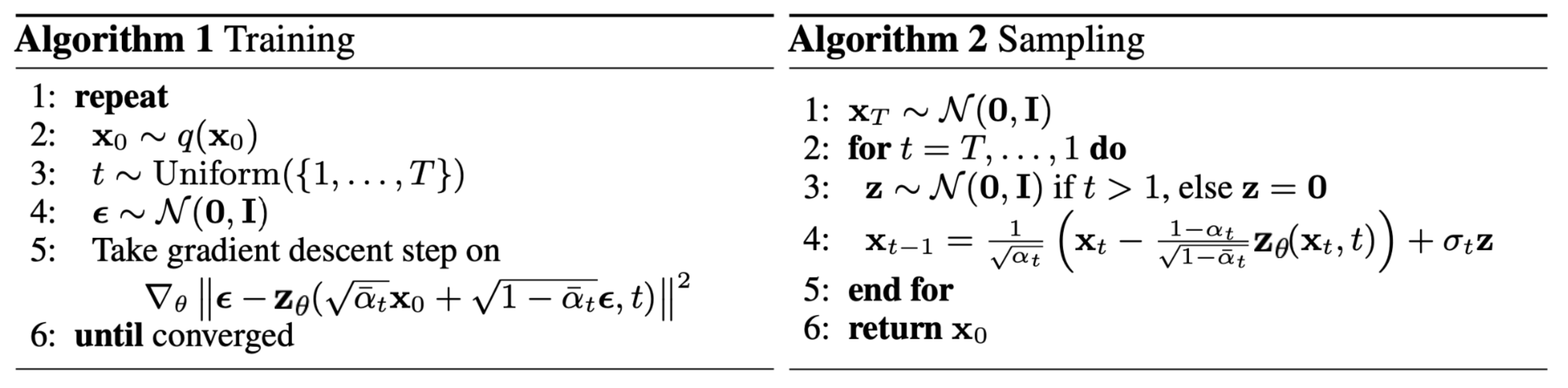Fig. 4. The training and sampling algorithms in DDPM (Image source: Ho et al. 2020)

Connection with noise-conditioned score networks (NCSN)

Song & Ermon (2019) proposed a score-based generative modeling method where samples are produced via Langevin dynamics using gradients of the data distribution estimated with score matching. The score of each sample $$\mathbf{x}$$’s density probability is defined as its gradient $$\nabla_{\mathbf{x}} \log p(\mathbf{x})$$. A score network $$s_\theta: \mathbb{R}^D \to \mathbb{R}^D$$ is trained to estimate it. To make it scalable with high-dimensional data in the deep learning setting, they proposed to use either denoising score matching (add a pre-specified small noise to the data; Vincent, 2011) or slided score matching (use random projections; Yang et al., 2019).

Recall that Langevin dynamics can sample data points from a probability density distribution using only the score $$\nabla_{\mathbf{x}} \log p(\mathbf{x})$$ in an iterative process.

However, according to the manifold hypothesis, most of the data is expected to concentrate in a low dimensional manifold, even though the observed data might look only arbitrarily high-dimensional. It brings a negative effect on score estimation since the data points cannot cover the whole space. In regions where data density is low, the score estimation is less reliable. After adding a small Gaussian noise to make the perturbed data distribution cover the full space $$\mathbb{R}^D$$, the training of the score estimator network becomes more stable. Song & Ermon (2019) improved it by perturbing the data with the noise of different levels and train a noise-conditioned score network to jointly estimate the scores of all the perturbed data at different noise levels.

The schedule of increasing noise levels resembles the forward diffusion process.

Parameterization of $$\beta_t$$

The forward variances are set to be a sequence of linearly increasing constants in Ho et al. (2020), from $$\beta_1=10^{-4}$$ to $$\beta_T=0.02$$. They are relatively small compared to the normalized image pixel values between $$[-1, 1]$$. Diffusion models in their experiments showed high-quality samples but still could not achieve competitive model log-likelihood as other generative models.

Nichol & Dhariwal (2021) proposed several improvement techniques to help diffusion models to obtain lower NLL. One of the improvements is to use a cosine-based variance schedule. The choice of the scheduling function can be arbitrary, as long as it provides a near-linear drop in the middle of the training process and subtle changes around $$t=0$$ and $$t=T$$.

$\beta_t = \text{clip}(1-\frac{\bar{\alpha}_t}{\bar{\alpha}_{t-1}}, 0.999) \quad\bar{\alpha}_t = \frac{f(t)}{f(0)}\quad\text{where }f(t)=\cos\Big(\frac{t/T+s}{1+s}\cdot\frac{\pi}{2}\Big)$

where the small offset $$s$$ is to prevent $$\beta_t$$ from being too small when close to $$t=0$$.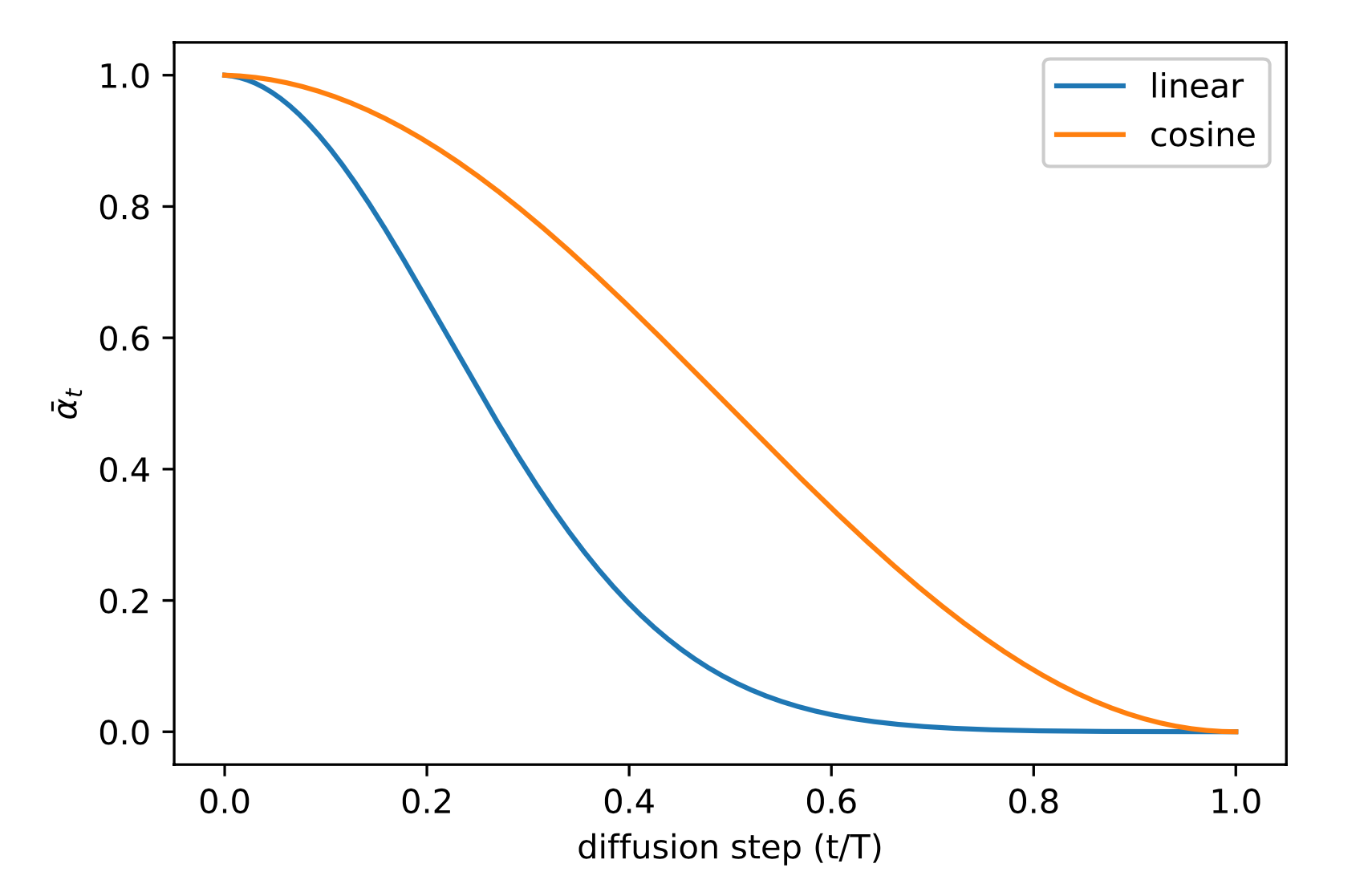Fig. 5. Comparison of linear and cosine-based scheduling of $$\beta_t$$ during training. (Image source: Nichol & Dhariwal, 2021)

Parameterization of reverse process variance $$\boldsymbol{\Sigma}_\theta$$

Ho et al. (2020) chose to fix $$\beta_t$$ as constants instead of making them learnable and set $$\boldsymbol{\Sigma}_\theta(\mathbf{x}_t, t) = \sigma^2_t \mathbf{I}$$ , where $$\sigma_t$$ is not learned but set to $$\beta_t$$ or $$\tilde{\beta}_t = \frac{1 - \bar{\alpha}_{t-1}}{1 - \bar{\alpha}_t} \cdot \beta_t$$. Because they found that learning a diagonal variance $$\boldsymbol{\Sigma}_\theta$$ leads to unstable training and poorer sample quality.

Nichol & Dhariwal (2021) proposed to learn $$\boldsymbol{\Sigma}_\theta(\mathbf{x}_t, t)$$ as an interpolation between $$\beta_t$$ and $$\tilde{\beta}_t$$ by model predicting a mixing vector $$\mathbf{v}$$ :

$\boldsymbol{\Sigma}_\theta(\mathbf{x}_t, t) = \exp(\mathbf{v} \log \beta_t + (1-\mathbf{v}) \log \tilde{\beta}_t)$

However, the simple objective $$L_\text{simple}$$ does not depend on $$\boldsymbol{\Sigma}_\theta$$ . To add the dependency, they constructed a hybrid objective $$L_\text{hybrid} = L_\text{simple} + \lambda L_\text{VLB}$$ where $$\lambda=0.001$$ is small and stop gradient on $$\boldsymbol{\mu}_\theta$$ in the $$L_\text{VLB}$$ term such that $$L_\text{VLB}$$ only guides the learning of $$\boldsymbol{\Sigma}_\theta$$. Empirically they observed that $$L_\text{VLB}$$ is pretty challenging to optimize likely due to noisy gradients, so they proposed to use a time-averaging smoothed version of $$L_\text{VLB}$$ with importance sampling.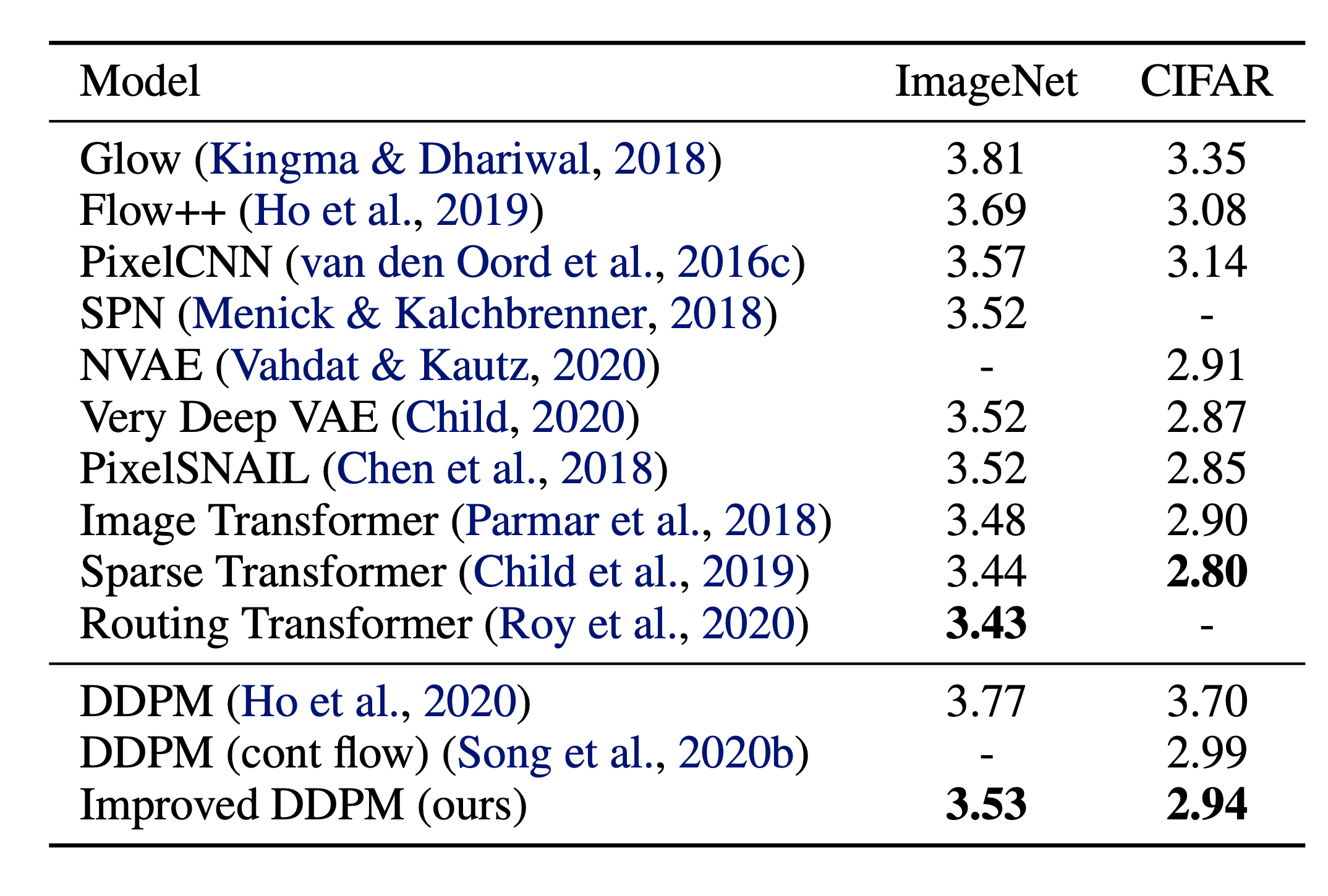Fig. 6. Comparison of negative log-likelihood of improved DDPM with other likelihood-based generative models. NLL is reported in the unit of bits/dim. (Image source: Nichol & Dhariwal, 2021)

Speed up Diffusion Model Sampling

It is very slow to generate a sample from DDPM by following the Markov chain of the reverse diffusion process, as $$T$$ can be up to one or a few thousand steps. One data point from Song et al. 2020: “For example, it takes around 20 hours to sample 50k images of size 32 × 32 from a DDPM, but less than a minute to do so from a GAN on an Nvidia 2080 Ti GPU.”

One simple way is to run a strided sampling schedule (Nichol & Dhariwal, 2021) by taking the sampling update every $$\lceil T/S \rceil$$ steps to reduce the process from $$T$$ to $$S$$ steps. The new sampling schedule for generation is $$\{\tau_1, \dots, \tau_S\}$$ where $$\tau_1 < \tau_2 < \dots <\tau_S \in [1, T]$$ and $$S < T$$.

For another approach, let’s rewrite $$q_\sigma(\mathbf{x}_{t-1} \vert \mathbf{x}_t, \mathbf{x}_0)$$ to be parameterized by a desired standard deviation $$\sigma_t$$ according to the nice property:

\begin{aligned} \mathbf{x}_{t-1} &= \sqrt{\bar{\alpha}_{t-1}}\mathbf{x}_0 + \sqrt{1 - \bar{\alpha}_{t-1}}\mathbf{z}_{t-1} \\ &= \sqrt{\bar{\alpha}_{t-1}}\mathbf{x}_0 + \sqrt{1 - \bar{\alpha}_{t-1} - \sigma_t^2} \mathbf{z}_t + \sigma_t\mathbf{z} \\ &= \sqrt{\bar{\alpha}_{t-1}}\mathbf{x}_0 + \sqrt{1 - \bar{\alpha}_{t-1} - \sigma_t^2} \frac{\mathbf{x}_t - \sqrt{\bar{\alpha}_t}\mathbf{x}_0}{\sqrt{1 - \bar{\alpha}_t}} + \sigma_t\mathbf{z} \\ q_\sigma(\mathbf{x}_{t-1} \vert \mathbf{x}_t, \mathbf{x}_0) &= \mathcal{N}(\mathbf{x}_{t-1}; \sqrt{\bar{\alpha}_{t-1}}\mathbf{x}_0 + \sqrt{1 - \bar{\alpha}_{t-1} - \sigma_t^2} \frac{\mathbf{x}_t - \sqrt{\bar{\alpha}_t}\mathbf{x}_0}{\sqrt{1 - \bar{\alpha}_t}}, \sigma_t^2 \mathbf{I}) \end{aligned}

Recall that in $$q(\mathbf{x}_{t-1} \vert \mathbf{x}_t, \mathbf{x}_0) = \mathcal{N}(\mathbf{x}_{t-1}; \tilde{\boldsymbol{\mu}}(\mathbf{x}_t, \mathbf{x}_0), \tilde{\beta}_t \mathbf{I})$$, therefore we have:

$\tilde{\beta}_t = \sigma_t^2 = \frac{1 - \bar{\alpha}_{t-1}}{1 - \bar{\alpha}_t} \cdot \beta_t$

Let $$\sigma_t^2 = \eta \cdot \tilde{\beta}_t$$ such that we can adjust $$\eta \in \mathbb{R}^+$$ as a hyperparameter to control the sampling stochasticity. The special case of $$\eta = 0$$ makes the sampling process deterministic. Such a model is named the denoising diffusion implicit model (DDIM; Song et al., 2020). DDIM has the same marginal noise distribution but deterministically maps noise back to the original data samples.

During generation, we only sample a subset of $$S$$ diffusion steps $$\{\tau_1, \dots, \tau_S\}$$ and the inference process becomes:

$q_{\sigma, \tau}(\mathbf{x}_{\tau_{i-1}} \vert \mathbf{x}_{\tau_t}, \mathbf{x}_0) = \mathcal{N}(\mathbf{x}_{\tau_{i-1}}; \sqrt{\bar{\alpha}_{t-1}}\mathbf{x}_0 + \sqrt{1 - \bar{\alpha}_{t-1} - \sigma_t^2} \frac{\mathbf{x}_{\tau_i} - \sqrt{\bar{\alpha}_t}\mathbf{x}_0}{\sqrt{1 - \bar{\alpha}_t}}, \sigma_t^2 \mathbf{I})$

While all the models are trained with $$T=1000$$ diffusion steps in the experiments, they observed that DDIM ($$\eta=0$$) can produce the best quality samples when $$S$$ is small, while DDPM ($$\eta=1$$) performs much worse on small $$S$$. DDPM does perform better when we can afford to run the full reverse Markov diffusion steps ($$S=T=1000$$). With DDIM, it is possible to train the diffusion model up to any arbitrary number of forward steps but only sample from a subset of steps in the generative process.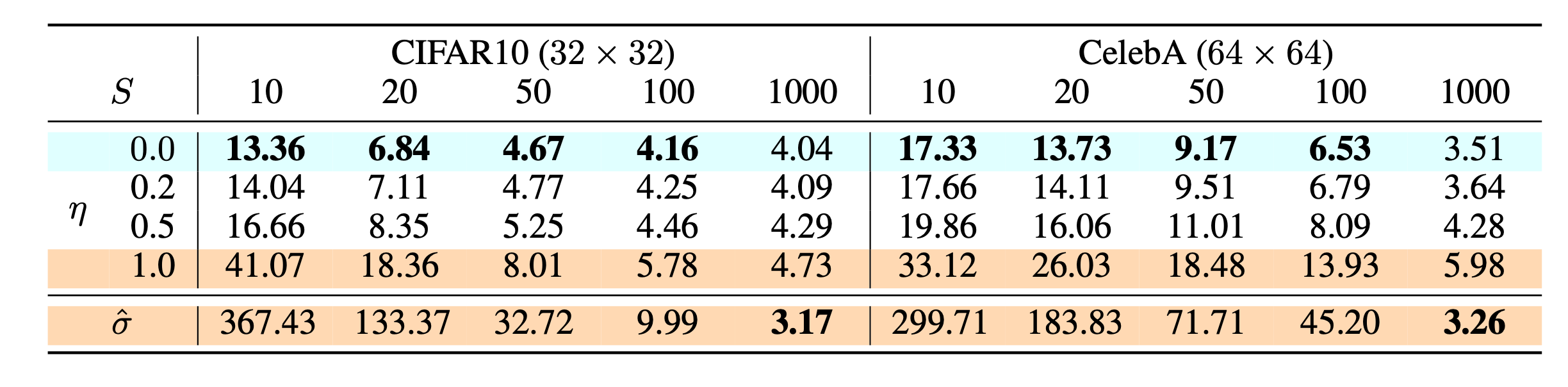Fig. 7. FID scores on CIFAR10 and CelebA datasets by diffusion models of different settings, including $$\color{cyan}{\text{DDIM}}$$ ($$\eta=0$$) and $$\color{orange}{\text{DDPM}}$$ ($$\hat{\sigma}$$). (Image source: Song et al., 2020)

Compared to DDPM, DDIM is able to:

1. Generate higher-quality samples using a much fewer number of steps.
2. Have “consistency” property since the generative process is deterministic, meaning that multiple samples conditioned on the same latent variable should have similar high-level features.
3. Because of the consistency, DDIM can do semantically meaningful interpolation in the latent variable.

Conditioned Generation

While training generative models on ImageNet data, it is common to generate samples conditioned on class labels. To explicit incorporate class information into the diffusion process, Dhariwal & Nichol (2021) trained a classifier $$f_\phi(y \vert \mathbf{x}_t, t)$$ on noisy image $$\mathbf{x}_t$$ and use gradients $$\nabla_\mathbf{x} \log f_\phi(y \vert \mathbf{x}_t, t)$$ to guide the diffusion sampling process toward the target class label $$y$$. Their ablated diffusion model (ADM) and the one with additional classifier guidance (ADM-G) are able to achieve better results than SOTA generative models (e.g. BigGAN).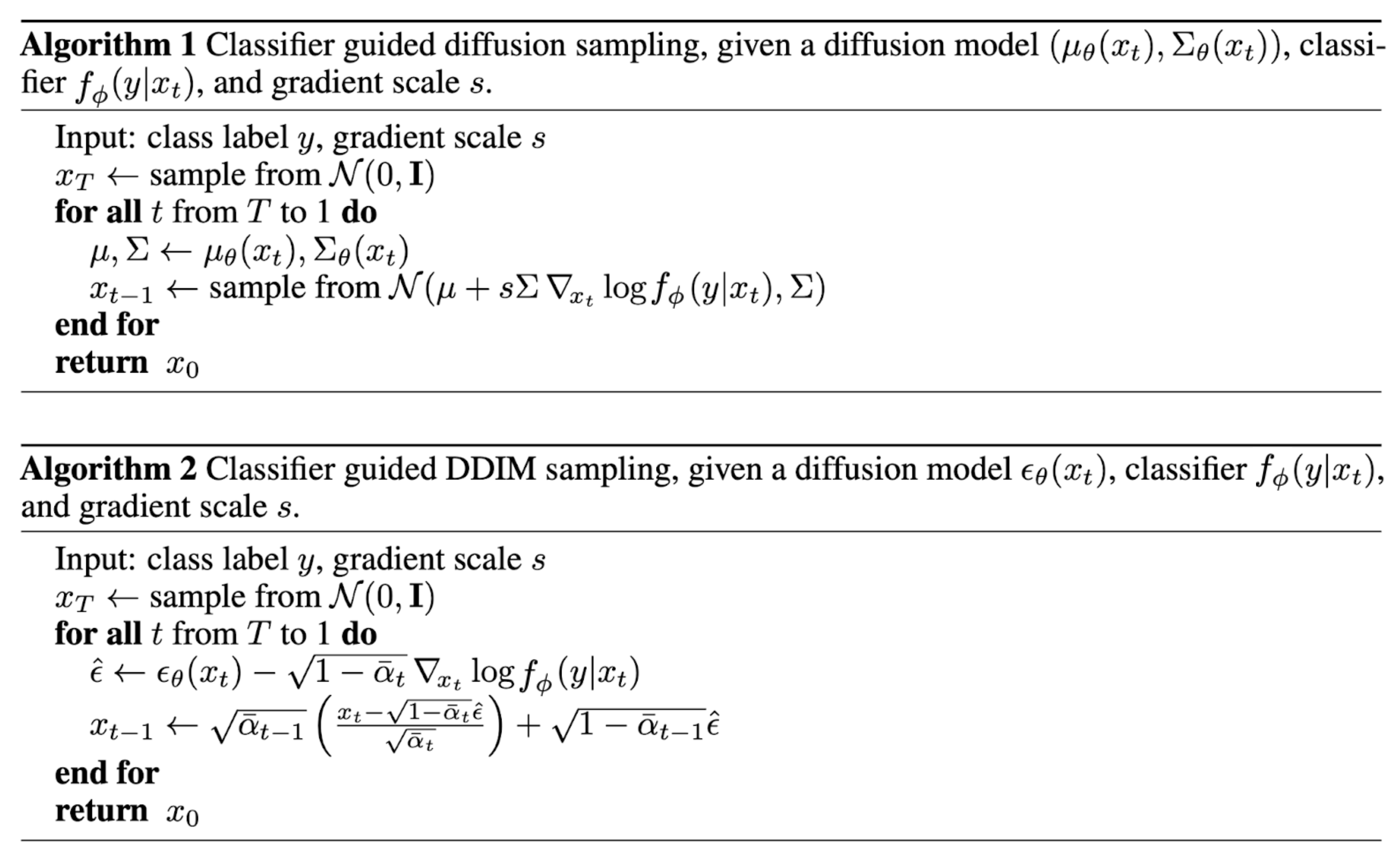Fig. 8. The algorithms use guidance from a classifier to run conditioned generation with DDPM and DDIM. (Image source: Dhariwal & Nichol, 2021])

Additionally with some modifications on the UNet architecture, Dhariwal & Nichol (2021) showed performance better than GAN with diffusion models. The architecture modifications include larger model depth/width, more attention heads, multi-resolution attention, BigGAN residual blocks for up/downsampling, residual connection rescale by $$1/\sqrt{2}$$ and adaptive group normalization (AdaGN).

Quick Summary

• Pros: Tractability and flexibility are two conflicting objectives in generative modeling. Tractable models can be analytically evaluated and cheaply fit data (e.g. via a Gaussian or Laplace), but they cannot easily describe the structure in rich datasets. Flexible models can fit arbitrary structures in data, but evaluating, training, or sampling from these models is usually expensive. Diffusion models are both analytically tractable and flexible

• Cons: Diffusion models rely on a long Markov chain of diffusion steps to generate samples, so it can be quite expensive in terms of time and compute. New methods have been proposed to make the process much faster, but the sampling is still slower than GAN.

Cited as:

@article{weng2021diffusion,
title   = "What are diffusion models?",
author  = "Weng, Lilian",
journal = "lilianweng.github.io/lil-log",
year    = "2021",
url     = "https://lilianweng.github.io/lil-log/2021/07/11/diffusion-models.html"
}

 Jascha Sohl-Dickstein et al. “Deep Unsupervised Learning using Nonequilibrium Thermodynamics.” ICML 2015.

 Max Welling & Yee Whye Teh. “Bayesian learning via stochastic gradient langevin dynamics.” ICML 2011.

 Yang Song & Stefano Ermon. “Generative modeling by estimating gradients of the data distribution.” NeurIPS 2019.

 Yang Song & Stefano Ermon. “Improved techniques for training score-based generative models.” NeuriPS 2020.

 Jonathan Ho et al. “Denoising diffusion probabilistic models.” arxiv Preprint arxiv:2006.11239 (2020). [code]

 Jiaming Song et al. “Denoising diffusion implicit models.” arxiv Preprint arxiv:2010.02502 (2020). [code]

 Alex Nichol & Prafulla Dhariwal. “ Improved denoising diffusion probabilistic models” arxiv Preprint arxiv:2102.09672 (2021). [code]

 Praffula Dhariwal & Alex Nichol. “Diffusion Models Beat GANs on Image Synthesis.” arxiv Preprint arxiv:2105.05233 (2021). [code]